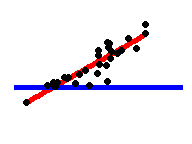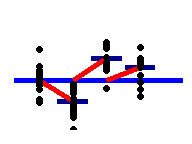# 常见的统计检验是线性模型

R 内置函数
R 中的等价线性模型

y 独立于 x
P：单样本 t 检验
N：Wilcoxon 符号秩检验
t.test(y)wilcox.test(y)
lm(y ~ 1)lm(signed_rank(y) ~ 1)

-（相同，但是它预测 y 的符号秩。）P：配对样本 t 检验
N：Wilcoxon 配对组检验
t.test(y1, y2, paired=TRUE)wilcox.test(y1, y2, paired=TRUE)
lm(y2 - y1 ~ 1)lm(signed_rank(y2 - y1) ~ 1)

-（相同，但是它预测 y2-y1 的符号秩。y ~ 连续变量 x
P：Pearson 相关系数
N：Spearman 相关系数
cor.test(x, y, method='Pearson')cor.test(x, y, method=Spearman')
lm(y ~ 1 + x)lm(rank(y) ~ 1 + rank(x))

-（相同，但是使用了 x 和 y 的y ~ 离散变量 x
P：双样本 t 检验
P：Welch t 检验
N：Mann-Whitney U 检验
t.test(y1, y2, var.equal=TRUE)t.test(y1, y2, var.equal=FALSE)wilcox.test(y1, y2)
lm(y ~ 1 + G2)Agls(y ~ 1 + G2, weights=...B)Alm(signed_rank(y) ~ 1 + G2)A

N > 11

-（相同，但是每一组用不同的方差，而不是用着共同的方差。）
-（相同，不过这个预测了 y 的符号秩。）P：单因素方差分析（one-way ANOVA）
N：Kruskall-Wallis 检验
aov(y ~ group)kruskal.test(y ~ group)
lm(y ~ 1 + G2 + G3 + .. + Gn)Alm(rank(y) ~ 1 + G2 + G3 + .. + Gn)A

-（相同，不过这个预测了 y 的。）P：单因素协变量分析（one-way ANCOVA）
aov(y ~ group + x)
lm(y ~ 1 + G2 + G3 + ... + Gn + x)A
-（相同，不过加上了 x 的斜率。）P：双因素方差分析（two-way ANOVA）
aov(y ~ group * sex)
lm(y ~ 1+G2+G3+...+Gn+ S2+S3+...+Sk+ G2*S2+G3*S3+...+Gn*Sk)

（待绘制）

N：卡方检验
chisq.test(groupXsex_table)

glm(y ~ 1+G2+G3+...+Gn+ S2+S3+...+Sk+ G2*S2+G3*S3+...+Gn*Sk, family=...)A

N：拟合优度检验
chisq.test(y)
glm(y ~ 1 + G2 + G3 + ... + Gn, family=...)A
（和单因素方差分析一致，建议查阅卡方检验笔记。）